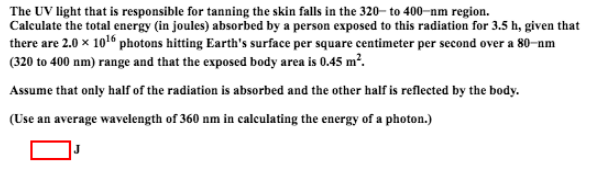# Problem: The UV light that is responsible for tanning the skin falls in the 320-to 400-nm region. Calculate the total energy (in joules) absorbed by a person exposed to this radiation for 3.5 h, given that there are 2.0 x 1016 photons hitting Earth's surface per square centimeter per second over a 80-nm (320 to 400 nm) range and that the exposed body area is 0.45 m2. Assume that only half of the radiation is absorbed and the other half is reflected by the body. (Use an average wavelength of 360 nm in calculating the energy of a photon.)

###### FREE Expert Solution
94% (78 ratings)###### Problem Details

The UV light that is responsible for tanning the skin falls in the 320-to 400-nm region. Calculate the total energy (in joules) absorbed by a person exposed to this radiation for 3.5 h, given that there are 2.0 x 1016 photons hitting Earth's surface per square centimeter per second over a 80-nm (320 to 400 nm) range and that the exposed body area is 0.45 m2

Assume that only half of the radiation is absorbed and the other half is reflected by the body.

(Use an average wavelength of 360 nm in calculating the energy of a photon.)